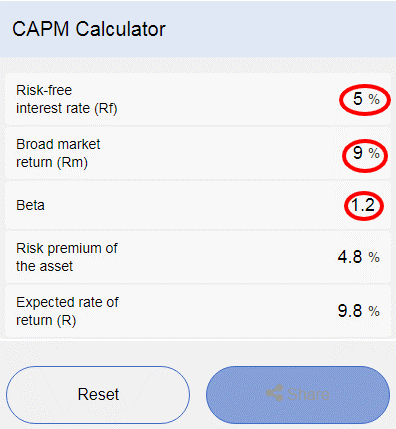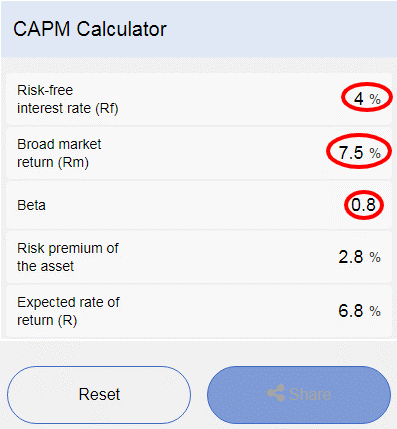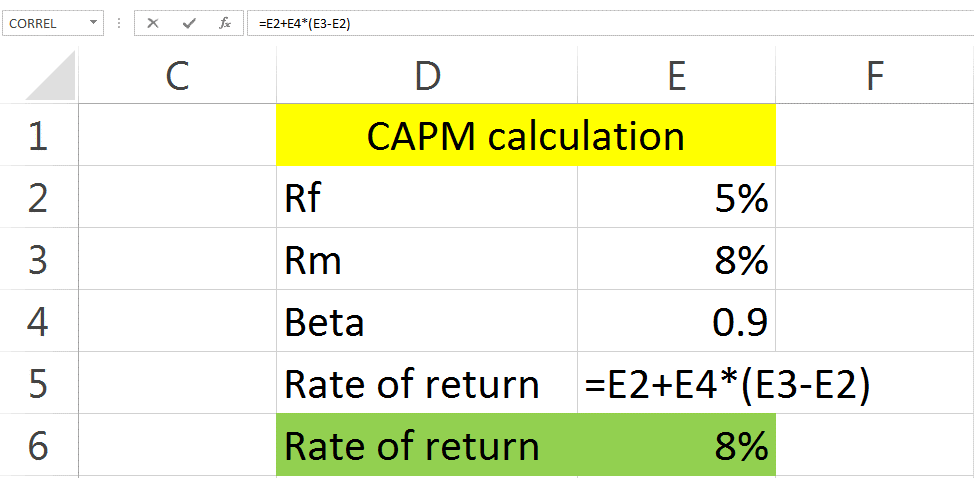##### Formula and Execution
 Risk-free interest rate (RF) The rate of return on government bonds is called the risk free rate. Broad market return ( Rm) The return on the market portfolio of investors is called a market return. Beta The fluctuation in stock prices and returns against the market prices and returns is called Beta. The risk premium of the asset The difference between market return and the risk-free rate is called the risk premium of the asset. Expected rate of return (R) The expected value of your investment is called the expected rate of return.

# Capital Asset Pricing Model (CAPM Calculator)

Historically the stock market is volatile, and there is up and down in stock prices daily. The fluctuation in share prices shows the riskiness of the stock market. This fluctuation in stock prices compels the investors to think about the high rate of return on share prices, which cover the risk. Stock market experts use different models to calculate the rate of return on the stock; one such model is the capital asset, pricing model. Calculating the rate of return on the stock is a challenging and time-consuming task. To avoid time consumption and increase work rate, CalculatorBeast Created different tools which assist financial analyst and stock market brokers in calculating things swiftly and correctly. One such tool is the CAPM calculator.

Why is the CAPM calculator used?

Calculating the rate of return manually has two main problems.

1. It consumes a lot of time and energy.
2. There is always a chance of error in manual calculation.

So to get rid of such problems, we use the CAPM calculator to calculate the rate of return quickly and precisely.

How can it help?

CAPM calculator is helpful in many ways such as:

• It saves your time through quick calculations.
• If you put correct values, then the chances of error in calculation is negligible.
• CAPM calculator will cost you nothing, while in return, the use of the CAPM calculator gives you the value of the rate of return on portfolio accurately and timely.

To whom it will help?

CAPM calculator is helpful for all those who are interested in the calculation of the expected rate of return on shares of the company. It can be useful to the following peoples.

• Researchers: Researchers can take help from the CAPM calculator in the calculation of the expected rate of return for their research papers.
• Students: Those students who are solving their assignments on the expected rate of return can take help from the CAPM calculator.
• Investors: The individual investors who want to invest their money in the stock market can also take help from the CAPM calculator while calculating the expected rate of returns
• Stock market brokers: The stock market brokers can also use the CAPM calculator for calculation of the rate of return on shares.

## Steps Involved in the CAPM Calculator

Three steps are required in CAPM calculator:

1. Risk-free rate
2. Market return
3. Beta

Put the values of the above terminologies in the CAPM calculator; you will get the value of the expected rate of return.

Example: An investor wants to invest money in the New York stock exchange. The risk-free rate on U.S treasury bonds is 5%, the market rate is 9% while stock beta is 1.2. Find the expected rate of return.

Solution:

Risk free rate = 5%

Market rate = 9%

Beta = 1.2

Rate of return = RF + beta (Rm- RF)

0.05 + 1.2 (0.09 – 0.05)

= 0.098 or 9.8%

Expected rate of return = 9.8%

The rate of return on the stock is higher than the market return. This is because the investor is investing his money in such securities whose risk is more than market risk. Higher the risk higher the returns and vice versa.

Note: The value of beta measures risk. Beta is systematic risk and is unavoidable. The market risk always lies between +1 and -1, and the stock beta is compared with the market beta. If the stock beta is greater than market beta, i.e., 1, the rate of return will be high and vice versa.

Calculating it manually takes time, and there is a chance of error. The easy way to find the rate of return is to put the values mentioned above in the CAPM calculator, and it will give you the expected rate of return as follow:## Formulas Used in the CAPM Calculator

Two formulas are used in the CAPM calculator:

• Risk premium formula: This formula is used to find the risk premium of an asset. the formula of risk premium is ( Market return - Risk-free rate )
• CAPM formula: This formula is used to find the expected rate of return on assets. The formula of CAPM is {Risk-free rate + Beta ( market return – Risk-free rate ) }

Example: An investor is willing to invest money in an XYZ company listed on the U.S stock exchange. The risk-free rate on U.S treasury bonds is 4%, and the market return is 7.5%. The Beta of the stock is 0.8. Find the risk premium and rate of return.

Solution:

Risk free rate = 4%

Market return = 7.5%

Beta = 0.8

Risk premium = (market return – risk-free rate)

= 7.5% - 4%

It means that the market is giving you an extra 3.5% on taking the risk.

CAPM = RF + beta (risk premium)

= 0.04 + 0.8 (0.035)

= 0.068 or 6.8%

Rate of return (r) = 6.8%

Note: The rate of return on the stock is low as compare to the market, i.e., the stock rate of return is 6.8% while the market is giving you a 7.5 % return. The reason behind the low stock return is that that stock beta is less as compared to market beta, so low-risk low returns.

You can solve this question on the CAPM calculator quickly as follow:Note: Some financial analyst considers (Market return – risk-free rate) as risk premium while others contemplate (beta*(market return – risk-free rate)) as a market risk premium. There is no effect on the final value of the expected rate of return in both cases. We use the first formula in solving the question manually. In the CAPM calculator, the following formula of risk premium is used. There is no effect on the final values of the expected rate of return, i.e., 6.8% in both cases.

## FAQS

### Why is the capital asset pricing model used?

The capital asset pricing model explains the relationship between systematic risk, i.e., beta and expected returns. CAPM is one of the most used and reliable models for pricing stock market securities.

### What is the systematic and unsystematic risk?

Systematic risk: The risk which cannot be diversified .i.e. you cannot minimize systematic uncertainty. The value of BETA measures systematic risk.

Examples: inflation, interest rate, exchange rate fluctuation, tax reforms, overall downfall in the economy, political situation, etc. You cannot minimize the effect of inflation or changes in interest rates because it is affecting the whole economy of the country, not just a single industry. If you are confused about the manual calculation of inflation, then you can refer to our inflation-calculator. It gives you calculations of inflation correctly and timely.

Unsystematic risk: The risk can be diversified, i.e., the risk can be minimized.

Examples: Business risk, financing risk, liquidity risk, etc. All these risks affect the single industry, not the whole economy. Business risk means that a company has problems in its operations, so investors can easily switch on to an operationally strong industry.

Following are the pros of capital asset pricing model

• CAPM is simple and easy to use.
• It takes systematic and unsystematic risk into account.
• It eliminates the unsystematic risk.

• According to the CAPM model, the risk-free rate will remain constant, but in actual, the risk-free rate changes daily.
• According to CAPM, the Market return will never be negative, but it is not true because market return can be negative.
• According to CAPM, an individual investor can lend and borrow money on a risk-free rate, but this is not true as only governments are considered to be immune to default risk. So an individual always bears the default risk.

### Why does most stock have a positive beta?

Beta compares the risk and returns on the share. The stock market is very volatile, and most of the time, the value of Beta changes frequently. In general, the majority of the stock prices move in the same direction as the trend of the market. In such situations, the stock prices and market prices have a positive correlation; that's why we see the majority of the stock have a positive beta.

### What is a good CAPM?

If the estimated rate of return is higher than the market return, then it is a good CAPM and vice versa. The estimated rate of return will always be high from market return if the stock beta is above 1.

### Why do financial managers have some difficulty applying the CAPM in business decision making?

CAPM is based on some very unrealistic assumptions, while financial managers have a realistic view of the stock market. The stock market is demanding something else while CAPM is giving it unrealistic values. So when the market conditions do not agree with the values of CAPM, that’s where financial managers face problems while using CAPM.

### What is systematic risk in CAPM?

The value of BETA represents a systematic risk in CAPM, and it shows the relationship between risk and return.

### What does negative Beta in CAPM mean?

A negative beta means that the stock prices are moving in the opposite direction to that of market prices, i.e., when the market prices fall the stock price of individual investor rise and vice versa.

### Why is CAPM better than the dividend discount model?

CAPM takes systematic and unsystematic risks into account, while the dividend discount model does not take the systematic risk in the calculation of the rate of return. That’s why investors prefer CAPM over DDM.

### Can Beta be zero?

Government securities such as government bonds are risk-free, so the Beta of such securities is zero.

### Does CAPM include dividends?

CAPM is based on three crucial factors i.e.

• Risk-free rate
• Beta
• Market return

So CAPM does not include dividends in its calculations.

### How do you use CAPM to value stock?

One of the most important formulas in the valuation of the stock is the dividend discount model. We use the dividend discount model to value stock. The formula of dividend discount model is {P = Dividend / Rate of return}. The rate of return used in DDM is taken from CAPM.

### How do you calculate WACC using CAPM?

WACC is the overall cost of capital, including both shareholders and debt holders. The formula of WACC is {Weight of debt*cost of debt + Weight of equity*cost of equity). The cost of capital used in the WACC formula is taken from CAPM. So that's how CAPM is used to find WACC. You can calculate WACC through the WACC calculator here, WACC-calculator.

### Is the CAPM cost of equity?

The cost of equity is the reward equity holders get in exchange for owning assets of the company. The formula used to find the cost of capital Is CAPM and Dividend discount model. So yes, CAPM is the cost of equity.

### Do you use levered or unlevered Beta in CAPM?

• Levered Beta: The Beta, which has the effect of capital structure, i.e., the levered Beta, has the effect of equity and debts. The formula of levered beta is {Unlevered beta*(1+ (1-tax)* (debt / equity)}.
• Unlevered Beta: The Beta, which does not affect the capital structure, i.e., unlevered Beta, has no effect of debts. The formula of unlevered beta is (levered beta / 1+ {(1-tax) (debt / equity)}.

The Beta used in CAPM is levered Beta.

### What is the difference between CAPM and WACC?

WACC stands for the weighted average cost of capital, while CAPM stands for the capital asset pricing model.

WACC is the cost that a company is expecting to pay it for all its security holders, including shareholders and debt holders.CAPM is the cost that a company is hoping to pay it to shareholders only.

### Hoe does debt affect Beta?

If a company finances its assets with the debt, it exposes the company to default risk. If a company is risky, then its Beta will be more. So the more company finance its assets with the debt, the more will be company beta.

### How to calculate CAPM in excel?

There is no specific excel function to calculate CAPM, but we can figure it out manually in excel as follow:

1. Enter the Risk-Free Interest Rate in cell E2.
2. Enter the Broad Market Return in cell E3.
3. Enter the "Beta" in cell E4.
4. Make the formula "E2+E4*(E3-E2)"
5. You will get Rate of Return in E6.### Is CAPM the discount rate?

Yes, you can use CAPM as a discount rate for the derivation of different financial values.

### What is the beta formula used in CAPM?

The Beta used in CAPM is a levered beta, and the formula of levered Beta is {unlevered beta*(1+ (1-tax)*(debt/equity)}.

### Is CAPM a single factor model?

CAPM is a single factor model because it depends on a single factor, i.e., risk. According to CAPM risk and return move head to head, if the financial securities are risky, it will yield more return and vice versa.

### What is the market portfolio in CAPM?

If an investor invests in marketable securities of companies A, B, and C. This is called the market portfolio of an investor. According to CAPM, an investor will make a portfolio comprises of all the possible financial securities. In this way, an investor will diversify his unsystematic risk and is only exposed to systematic risk.

Example: There are four companies A, B, C, and D. these companies are offering different types of financial securities such as shares, bonds, commercial papers, etc. According to CAPM, an investor will make his portfolio comprises of all the financial securities of these companies. But this is practically impossible because an investor cannot invest in all securities due to the constraint of money and lacking the expertise to handle such an extensive portfolio.

### In which case, the risk-free rate will be more than the market return?

In a normal situation, market returns will always be higher than risk-free rates because investors who invest in market securities are taking additional risks. As a result, they will get the extra returns over and above the risk-free rate. But in a situation where the stock market completely crashes like the great depression of 1930 or 2008, the market return falls steeply. In such circumstances, there is a high chance that the risk-free rate will exceed market returns.

### What is the market return?

The return on the overall market portfolio, including all the assets, is called a market return. The formula of market return is (Capital gain rate + dividend yield).

• Capital gain rate: The extra amount you receive on the selling of a share is called a capital gain. While the (Current capital gain – previous capital gain / previous capital gain) is called the capital gain rate.
• Dividend yield: The distribution of profit by the company to its shareholders is called the dividend. While the (Dividend per share / current price of the stock) is called the dividend yield.

### What a beta above 1 one mean?

As we know that the market beta is 1. If the portfolio beta is above 1, then it means that the portfolio is more volatile or risky than the market and vice versa. If a portfolio beta is 1.5, then it means that the portfolio is 1.5 times more volatile than the market.

### Is a high beta a good sign?

Higher the Beta higher will be the volatility and risk of the stock. So yes, if we see from the returns perspective, then higher Beta is a good sign because it will yield higher returns. While if we see it from risk and volatility angle, then it's not good signs because the more volatile a stock, the more there is a chance of a decrease in share prices. The decline in share price means that an investor is losing his investment. Higher Beta is a relative term and depends on which perspective you are explaining it.

### What is the difference between market risk premium and equity risk premium?

• Market risk premium: The returns over and above the risk-free rate is called the market risk premium. The market risk premium is gain at the portfolio you held. The portfolio is the combination of all types of assets, including stock and corporate bonds. The formula of market risk premium is (Market return – risk-free rate).
• Equity risk premium: The returns investors get over and above risk-free rate is called the equity risk premium. The equity risk premium is only related to stock. The equity risk premium is usually higher than the market risk premium.